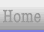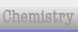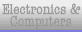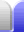# Angles, Triangles & Pythagoras

 Angle Types – acute, right, obtuse, straight, reflex, complementary, and supplementary. Triangles – scalene, isoceles, equilateral, and right Triangle centres – incentre (and incentre), circumcentre (and circumcircle), centroid (and barycentre), orthocentre. Pythagoras' Theorem – including ways to prove Pythagoras' theorem with algebra and visually. Pythagorean Triples – also Pythagorean quadruples and the famous Fermat's Last Theorem. Pythagoras & Trigonometry Revision – a few sample questions to see if the principles are understood, with pop-up answers.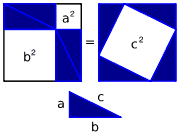Home Astronomy Chemistry Electronics & Computers Mathematics Physics Field Trips Turn on javascript for email link